Next: Free Motion of a Up: Dirac Equation Previous: Nonrelativistic Limit of the

# Constants of the Motion

Constants of the motions are those dynamic variables that commute with the Hamiltonian. For a free particle (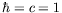)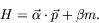(5.47)

With the usual definition of the orbital angular momentum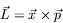(5.48)

we find that none of the components of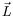commute with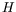. Thus the orbital angular momentum is not a constant of the motion. On the other hand we find that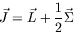(5.49)

with(5.50)

does commute withand thus is a constant of the motion.

We now prove this statement. To do this, we simply verify that this result is true for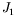. Clearly,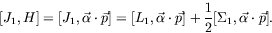(5.51)

For the first term in equation 5.51,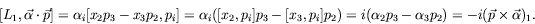(5.52)

For the second term in equation 5.51, we make use of the relationship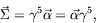(5.53)

where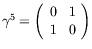. Using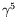we have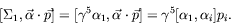(5.54)

Since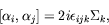(5.55)

we get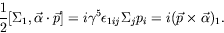(5.56)

Thus, we have shown that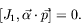(5.57)

By symmetry it now follows that quite generally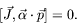(5.58)

As a consequence we have that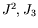andcan be simultaneously diagonalized.

The bonus embodied in the Dirac equation is the extra twofold degeneracy. This means that there must be another observable which commutes withand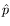, whose eigenvalues can be taken to distinguish the states. On inspection, we see that the operator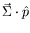commutes withand;is the unit vector pointing in the direction of the momentum,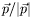. The spin'' component in the direction of the motion,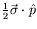, is therefore a good'' quantum number and can be used to label the solutions. We call this quantum number the helicity of the state.Next: Free Motion of a Up: Dirac Equation Previous: Nonrelativistic Limit of the
Douglas M. Gingrich (gingrich@ ualberta.ca)
2004-03-18